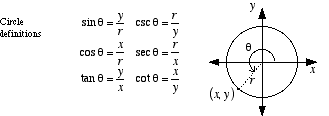index: click on a letter A B C D E F G H I J K L M N O P Q R S T U V W X Y Z A to Z index index: subject areas numbers & symbols sets, logic, proofs geometry algebra trigonometry advanced algebra & pre-calculus calculus advanced topics probability & statistics real world applications multimedia entrieswww.mathwords.com about mathwords website feedback

 Trig Functions Circular Functions The six functions sine, cosine, tangent, cosecant, secant, and cotangent. These functions can be defined several different ways. These include SOHCAHTOA definitions, circle definitions (below), and unit circle definitions.See also Inverse trig functions